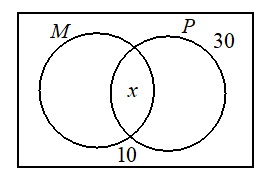In a class of 30 students, 10 take Mathematics, 15 take Physics, and 10 take neither. The number of students who take both mathematics and physics is

# In a class of 30 students, 10 take Mathematics, 15 take Physics, and 10 take neither. The number of students who take both mathematics and physics is1. A

15

2. B

5

3. C

3

4. D

2

Fill Out the Form for Expert Academic Guidance!l

+91

Live ClassesBooksTest SeriesSelf Learning

Verify OTP Code (required)

### Solution:

Total number of students $n\left(\mu \right)=30$

$n\left(M\right)=10$

$n\left(P\right)=15$

$n\left(M\cup P\right)=30-10=20$

Let  $n\left(M\cap P\right)=x$

$n\left(M\cup P\right)=n\left(M\right)+n\left(P\right)-n\left(M\cap P\right)$

$20=10+15-x$

$x=5$

## Related content

 Sarojini Naidu Biography Gender Discrimination Essay For Student Advantages and Disadvantages of Mobile Phones Essay Leave Application for Sister Marriage – Sister’s Wedding Application in Office/School World Post Office Day 2023 Indian Air Force Day Essay on Dussehra in Hindi for Students Check out! Top 10 high Paying Salary Courses After 12th Science World Cotton Day 2023 Leave Application for Job and for Office with Format and Samples+91

Live ClassesBooksTest SeriesSelf Learning

Verify OTP Code (required)